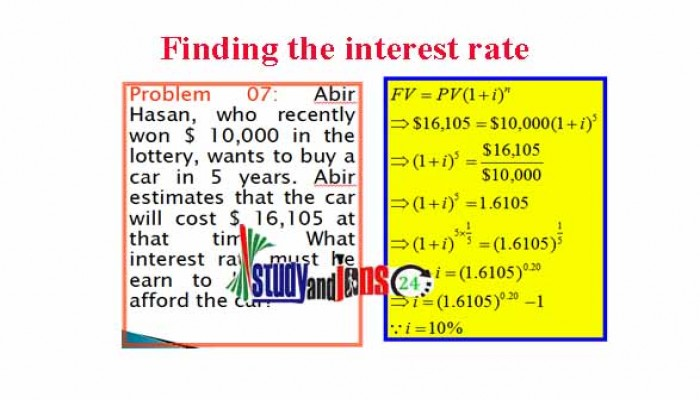Zulfiqar Hasan

Interest is a fixed charge on borrowed fund.

Simple interest refers to interest earned only on the original capital investment amount.

Compound interest refers to interest earned on both the initial capital investment and on the interest reinvested from prior periods.

By using time value of money concept, one can find out the rate of interest. One can use either Present Value formula or Future value formula. No need to use the logarithm.

Problem:

Abir Hasan, who recently won BDT10,000 in the lottery, wants to buy a car in 5 years. Abir estimates that the car will cost BDT16,105 at that time. What interest rate must he earn to be able to afford the car?

Solution:

So, i = 10%

In this process, you can find out the rate of interest without using logarithm.